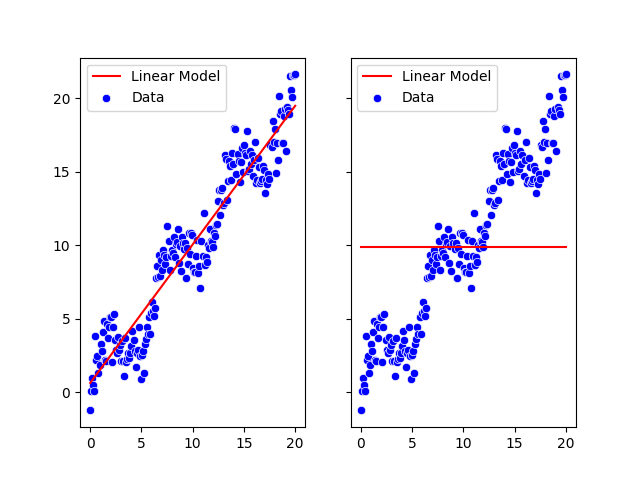# Same model running on GPU and CPU produce different results

Hi,
I have created a simple linear regression model. It runs fine with cpu but when I run the model on gpu it does not fit the model at all. Can some one find out any mistake I have done.

Here is a simple model definition. Its is just a test code nothing for production:

``````import torch
import torch.nn as nn
import seaborn as sns
import numpy as np
import matplotlib.pyplot as plt

np.random.seed(103)

def generate_1d_data():
features = np.linspace(0, 20, num=200)
targets = features + np.sin(features) * 2 + np.random.normal(size=features.shape)
return features, targets

class LinearRegressionModel(torch.nn.Module):
def __init__(self, input_dim, output_dim):
super(LinearRegressionModel, self).__init__()
self.linear = torch.nn.Linear(input_dim, output_dim)

def forward(self, x):
out = self.linear(x)
return out

def get_device(device='cuda'):
if device == 'cpu':

def get_prediction(model, device, inputs):
preds = model(inputs.to(device)).cpu().numpy()
return preds

def train(model, device, inputs, labels, epochs):
model.to(device)
for epoch in range(epochs):
print(f'Epoch {epoch}')
outputs = model(inputs.to(device))
loss = criterion(outputs, labels.to(device))
loss.backward()
optimizer.step()
return loss

X, y = generate_1d_data()
inputs = torch.tensor(X.reshape(-1, 1), dtype=torch.float32)
labels = torch.tensor(y.reshape(-1, 1), dtype=torch.float32)
criterion = nn.MSELoss()
``````

Running with CPU and GPU is as follows:

``````# Running with CPU
model = LinearRegressionModel(input_dim=1, output_dim=1)
optimizer = torch.optim.SGD(model.parameters(), lr=0.001)
device = get_device('cpu')

loss = train(model, device, inputs, labels, epochs=2000)
print("loss:", loss.data.cpu().numpy())
m = model.linear.weight.data.cpu().numpy()
b = model.linear.bias.data.cpu().numpy()
print("m(slope)=", m, "n(y-intercept)=", b)
predictions_cpu = get_prediction(model, device, inputs)

# Running with GPU
model = LinearRegressionModel(input_dim=1, output_dim=1)
optimizer = torch.optim.SGD(model.parameters(), lr=0.001)
device_gpu = get_device()

loss = train(model, device_gpu, inputs, labels, epochs=2000)
print("loss:", loss.data.cpu().numpy())
m = model.linear.weight.data.cpu().numpy()
b = model.linear.bias.data.cpu().numpy()
print("m(slope)=", m, "n(y-intercept)=", b)
predictions_gpu = get_prediction(model, device_gpu, inputs)
``````

But the results are so different not sure why left one CPU right one GPU. CPU training looks fine.Does any one see some mistake in the process. Thanks in advance.

In this sequence, you first grab the parameters while they’re on the CPU and stick them in the optimizer. Then you move the model to GPU in train.
I would recommend to either create the optimizer in train or do the moving right after instantiation (the latter is what I’d do, personally).

Best regards

Thomas

I have followed your suggestion and run the gpu code separately in a separate python interpreter as follows. But the effect is same.

``````import ....
.....
.....
def train(model, device, inputs, labels, epochs):
print(model.state_dict())
model.to(device)
optimizer = torch.optim.SGD(model.parameters(), lr=0.001)
print(model.state_dict())
for epoch in range(epochs):
model.train()
print(f'Epoch {epoch}')
outputs = model(inputs.to(device))
loss = criterion(outputs, labels.to(device))
loss.backward()
optimizer.step()
print(model.state_dict())
return loss

X, y = generate_1d_data()
inputs = torch.tensor(X.reshape(-1, 1), dtype=torch.float32)
labels = torch.tensor(y.reshape(-1, 1), dtype=torch.float32)
criterion = nn.MSELoss()

# Running with GPU
torch.cuda.empty_cache()
model = LinearRegressionModel(input_dim=1, output_dim=1)
device_gpu = get_device()

loss = train(model, device_gpu, inputs, labels, epochs=1000)
predictions_gpu = get_prediction(model, device_gpu, inputs)

sns.scatterplot(x=X, y=y, color='blue', label='Data')
sns.lineplot(x=X, y=predictions_gpu.ravel(), color='red', label='Linear Model')
plt.savefig('temp/training.png')
``````

But the prediction always produce constant result from gpu, not sure why.

``````predictions_gpu
array([[8.821195],
[8.821195],
[8.821195],
[8.821195],
[8.821195],
[8.821195],
[8.821195],
...
``````

But I have checked the parameter state has been changed from cpu to gpu. Initial state `OrderedDict([('linear.weight', tensor([[0.0323]])), ('linear.bias', tensor([0.9064]))])` to moving to gpu
`OrderedDict([('linear.weight', tensor([[0.0323]], device='cuda:0')), ('linear.bias', tensor([0.9064], device='cuda:0'))])` then final state `OrderedDict([('linear.weight', tensor([[145.3438]], device='cuda:0')), ('linear.bias', tensor([8.8212], device='cuda:0'))])`

Though I have given the correct input:

``````inputs
tensor([[ 0.0000],
[ 0.1005],
[ 0.2010],
[ 0.3015],
[ 0.4020],
[ 0.5025],
[ 0.6030],
[ 0.7035],
[ 0.8040],
[ 0.9045],
[ 1.0050],
.....
``````
``````pyplot.plot(inputs, predictions_gpu)
pyplot.scatter(inputs, labels)
``````

gives meNot sure why it isn’t the case for you, maybe you had an old copy of something somewhere?

1 Like

Ok, It looks like some problem with my gpu or something do not know why. I will check again. Thanks a lot for testing.